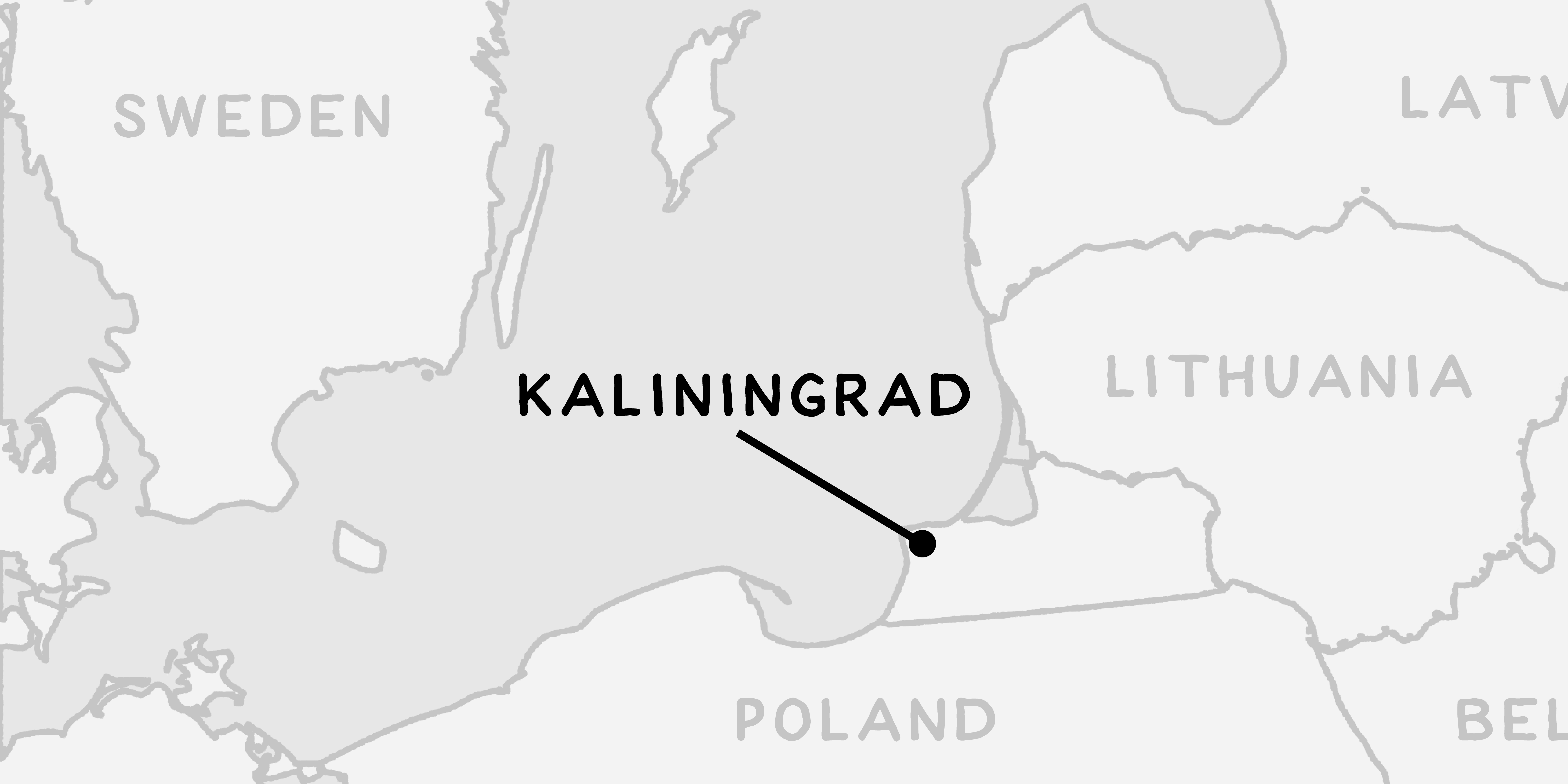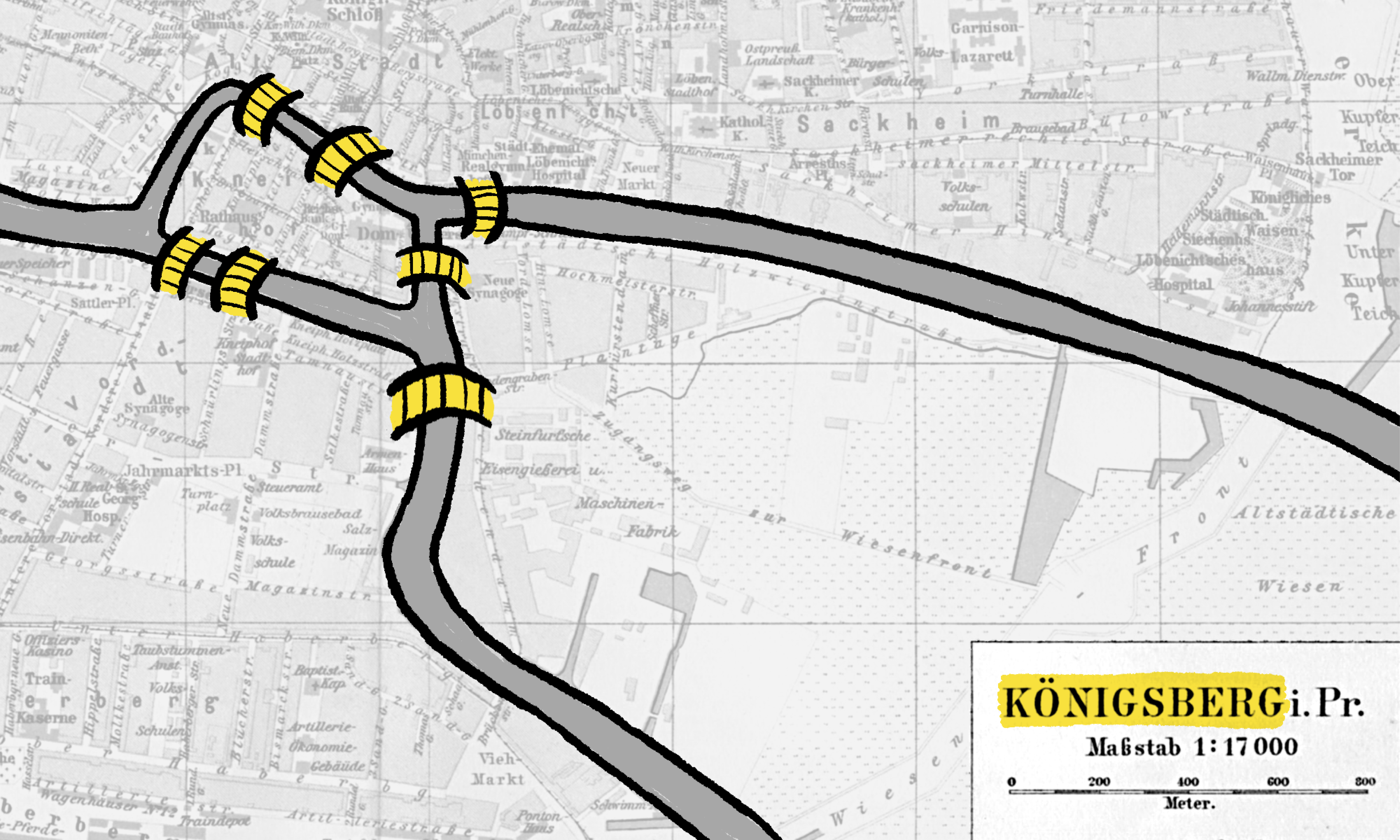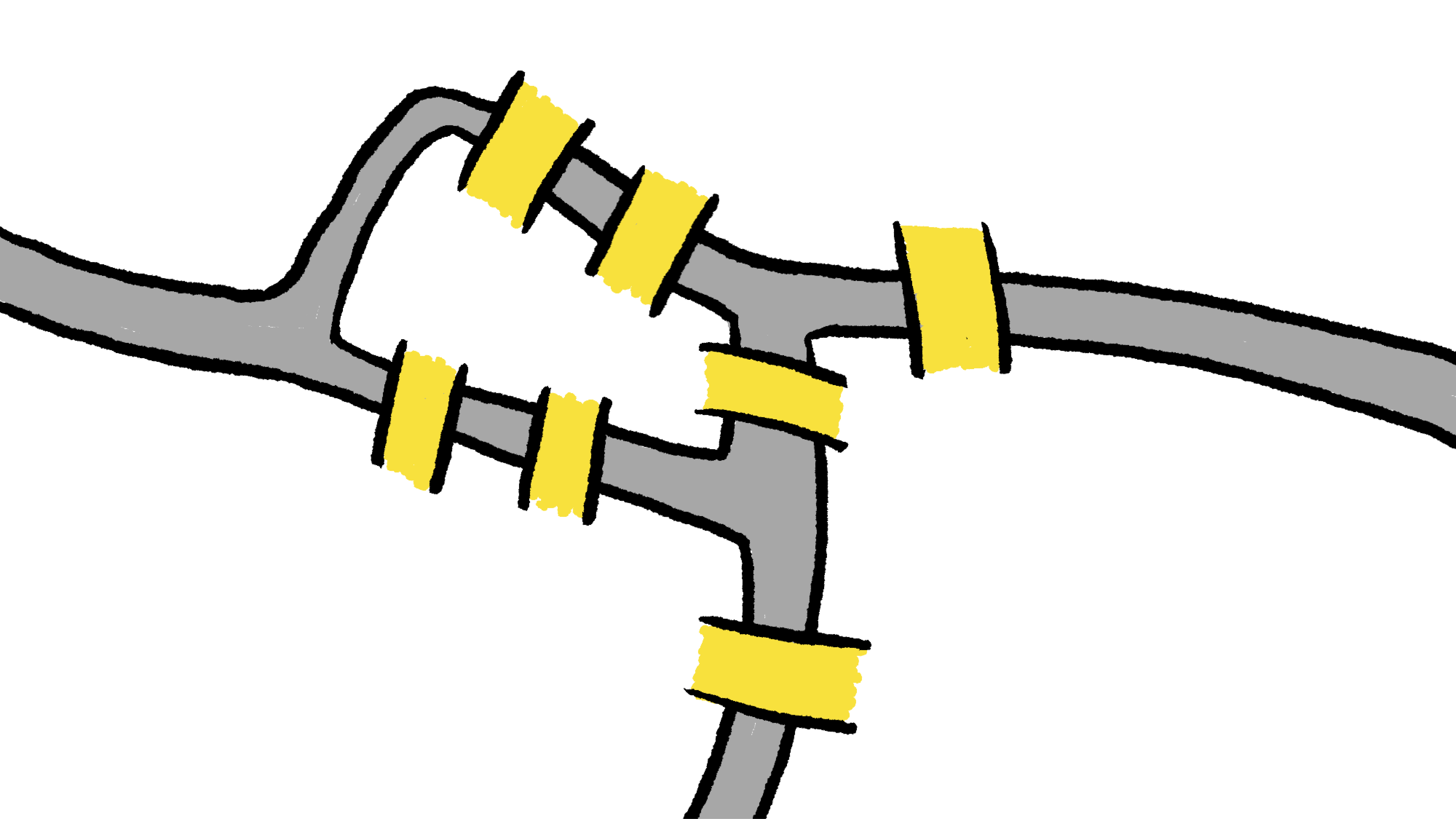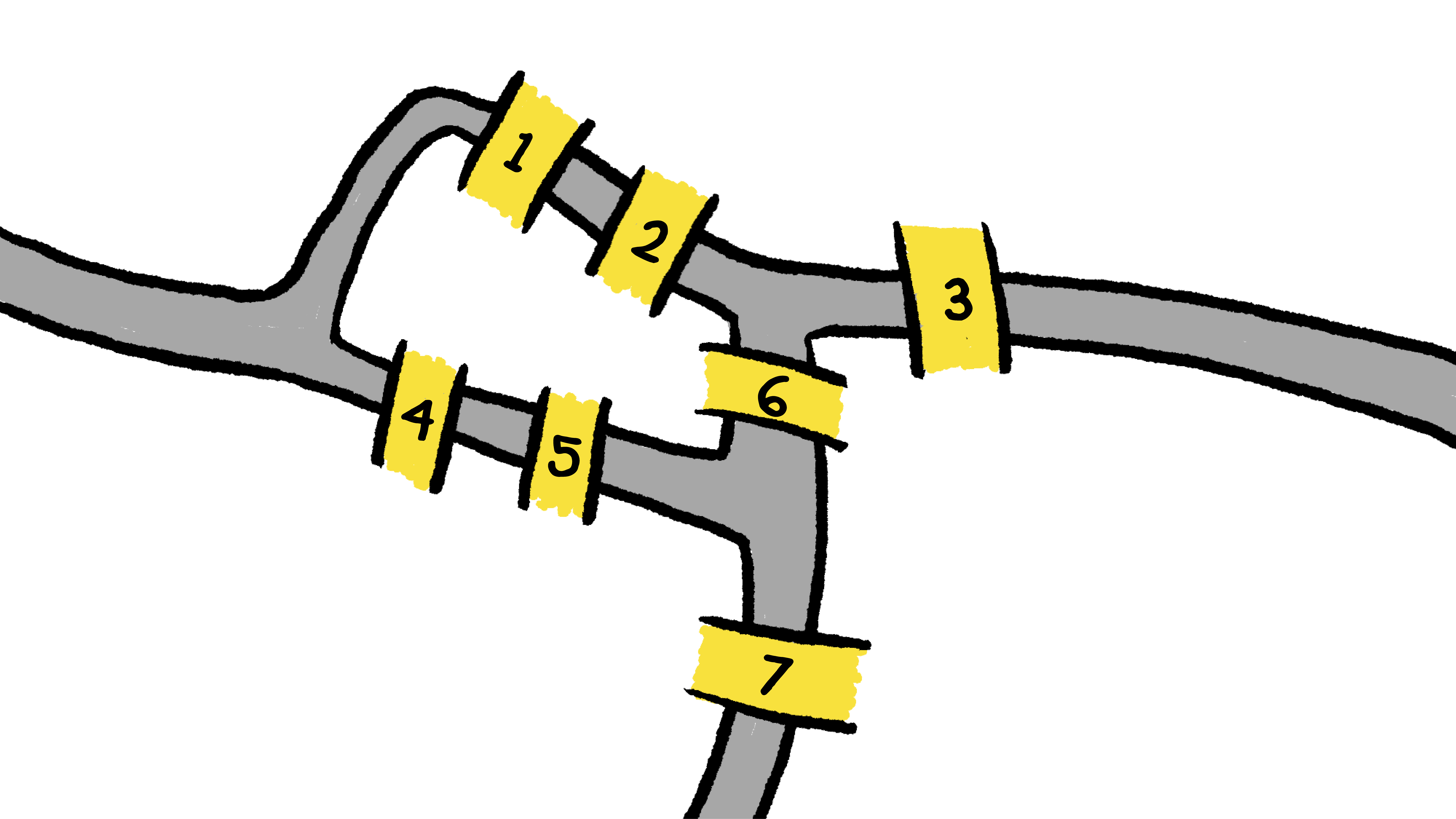### Computer Science Fundamentals

The Russian city of Kaliningrad lies about $30$ kilometers north of Poland, where the Pregolya River meets the Baltic Sea.The city has a famous place in mathematical history dating back to the eighteenth century when the city was named Königsberg and had seven bridges connecting two river islands in the heart of the city.In this exploration, you'll look at a classic puzzle about these seven bridges and create an impractical but interesting way to solve this puzzle. In the next exploration, we'll look at a more practical alternative!

# Searching for Solutions

This arrangement of Königsberg's seven bridges led people to ask a simple yes-or-no question: "Could you start anywhere in the city and cross every bridge exactly once?" (No swimming allowed!)

It's easy to attempt the puzzle and get stuck. Here is a path through the city that gets stuck after crossing four bridges.

# Searching for Solutions

Spoiler alert: It turns out that it was not possible to cross all seven bridges of Königsberg exactly once, even if you can start and end anywhere. (The interesting computer science enters the picture when you try to explain why you can't achieve this goal!)

Can you find a path that crosses six bridges before getting stuck? Remember, you're not allowed to cross any bridge twice.# Searching for Solutions

Even if you're allowed to start or end anywhere you want, you can't cross every bridge exactly once: you'll get stuck after at most six bridges.

Let's turn the problem on its head to make sure we understand it. If you're allowed to start anywhere, but you can't cross any single bridge twice, what is the fewest number of bridges you can cross before getting stuck?# Searching for Solutions

If you didn't know whether or not there was a solution to the puzzle of the bridges of Königsberg, you could try to find one by moving around randomly. Start at a random place, and then move across a random bridge until you succeed or get stuck.

Repeating this process over and over will always eventually find a path if there is a path to be found. However, repeatedly performing random explorations is not a good way for us to convince ourselves that no path exists. We can never be sure that there's not some solution we've missed by getting unlucky!

You might try to imagine a systematic way to explore the city, a method that ensures you will try every possible combination of bridges. One systematic way of exploring that computer scientists use is called depth-first search, but that's a topic for a different exploration.

# Searching for Solutions

In computer science, it's sometimes useful to look for a brute force solution. If you can describe what all the possible solutions look like, and if you can check what it means for a solution to be correct or valid, then just check all the possible solutions until you find one that works!

In the case of the bridges of Königsberg, we can number the seven bridges $1, 2, 3, 4, 5, 6,$ and $7.$Any solution to the puzzle is going to be some sequence of seven bridges that includes each of the seven bridges: that is, a permutation of the sequence $1, 2, 3, 4, 5, 6, 7.$

We can list each possible permutation, and then check each permutation in turn to see if that sequence of bridges represents a path that we can physically walk (without crossing a river). If even one of these sequences is possible to carry out, then it represents a solution to the puzzle.

Using this approach, how many bridge permutations would we need to check to be sure that there is no way to walk the seven bridges of Königsberg exactly once each?

# Searching for Solutions

Imagine that a computer can check $5040$ different permutations of bridges every second. (That would mean that it only takes a second to check all the permutations of bridges in Königsberg.)

A $2006$ study of Pittsburgh, Pennsylvania determined that the city had $446$ bridges.

If we use the same approach to see whether all the bridges in Pittsburgh can be crossed once without leaving any roadways, how long would that take?

# Searching for Solutions

It isn't too hard to figure out if any specific sequence of bridges represents a valid way through Königsberg. We can therefore solve the puzzle of the bridges of Königsberg by listing all the permutations of bridges and checking whether each combination works.

This brute force solution is not a particularly satisfying solution! It certainly doesn't help us once there are a couple more bridges. In the next quiz, we'll see how we can do much better.

For many important puzzles in computer science, the best way we know how to find an ideal solution is by brute force: listing all the possible solutions and then quickly checking each possible solution. (Maybe that's because there isn't any better way, but maybe that's because there is a better way and we just haven't thought of it yet! For most of the important problems involving brute force search, we don't know!)

Solving problems with brute force is an important tool in the computer science toolbox. However, brute force problem solving gets a lot harder when the solutions get only a little bit more complex.

×